# Solve twostep equations To solve twostep equations undo

• Slides: 12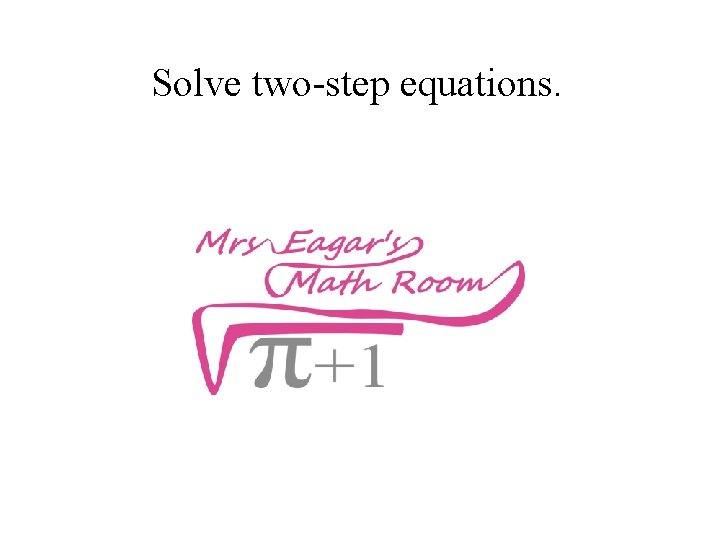Solve two-step equations.To solve two-step equations, undo the operations by working backwards. Example: Ask yourself, 1. What is the first thing we are doing to x? 2. What is the second thing? Recall the order of operations as you answer these questions. • Dividing by 2 • Subtracting 3 To undo these steps, do the opposite operations in opposite order.Use a DO-UNDO chart as a shortcut to answering the questions. In the table, write the opposite operations in the opposite order DO UNDO ÷ 2 +3 -3 · 2 1. Draw “the river” 2. Add 3 to both sides 3. 4. Follow the steps in the ‘undo’ column to isolate 5. the variable. 6. +3 +3 2 Simplify Clear the fraction Multiply both sides by 2 Simplify · = - 4· 2 Check your answer -4 – 3 = -7 x = -8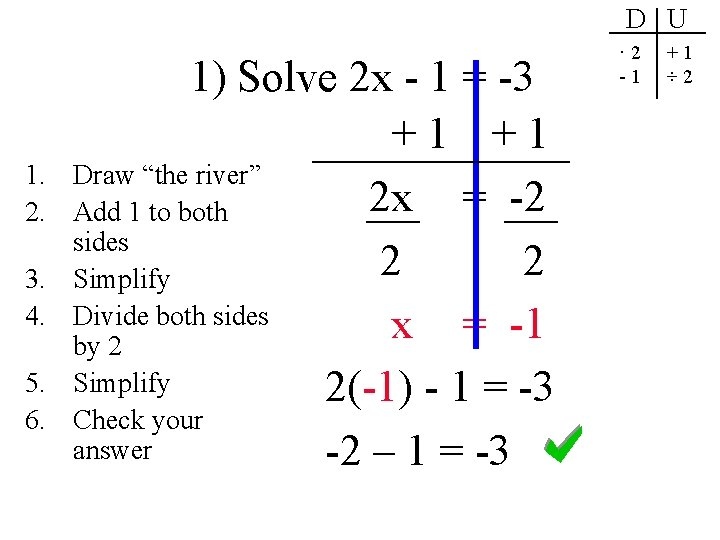D U 1. 2. 3. 4. 5. 6. 1) Solve 2 x - 1 = -3 +1 +1 Draw “the river” 2 x = -2 Add 1 to both sides 2 2 Simplify Divide both sides x = -1 by 2 Simplify 2(-1) - 1 = -3 Check your answer -2 – 1 = -3 · 2 -1 +1 ÷ 2D U 2) Solve 1. Draw “the river” 2. Add 4 to both sides 3. Simplify 4. Clear the fraction Multiply both sides by 3 5. Simplify 6. Check your answer ÷ 3 - 4 +4 +4 3· · 3 x = 36 12 – 4 = 8 +4 · 33) Solve 3 y – 1 = 8 1. y = 3 2. y = -3 3. y = 4. y = Answer Now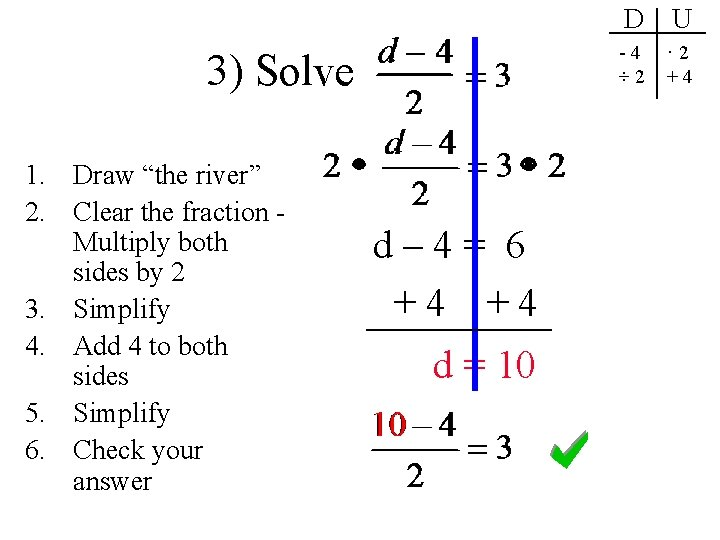3) Solve 1. Draw “the river” 2. Clear the fraction Multiply both sides by 2 3. Simplify 4. Add 4 to both sides 5. Simplify 6. Check your answer d– 4= 6 +4 +4 d = 10 D U -4 ÷ 2 · 2 +44) Solve 1. 2. 3. 4. d = -7 d = -19 d = -17 d = 17 Answer Now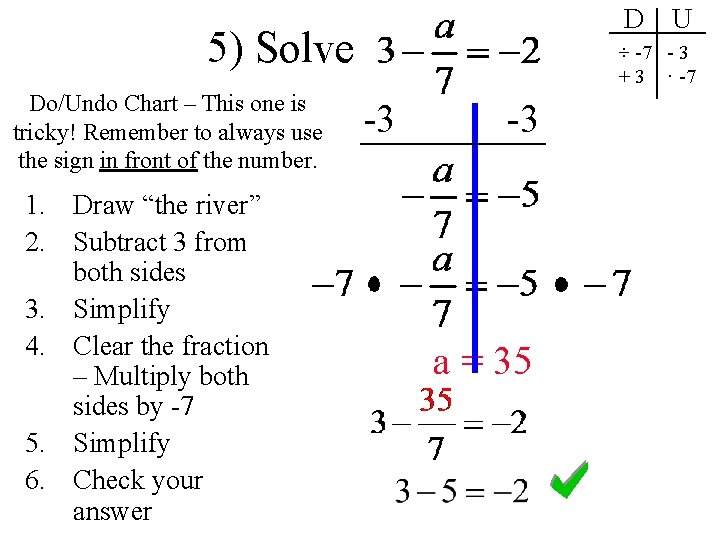D 5) Solve Do/Undo Chart – This one is tricky! Remember to always use the sign in front of the number. 1. Draw “the river” 2. Subtract 3 from both sides 3. Simplify 4. Clear the fraction – Multiply both sides by -7 5. Simplify 6. Check your answer U ÷ -7 - 3 + 3 · -7 -3 -3 a = 35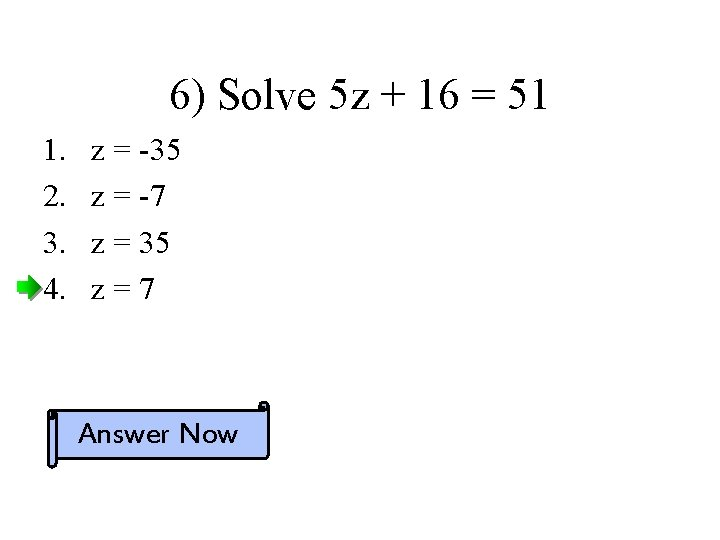6) Solve 5 z + 16 = 51 1. 2. 3. 4. z = -35 z = -7 z = 35 z=7 Answer Now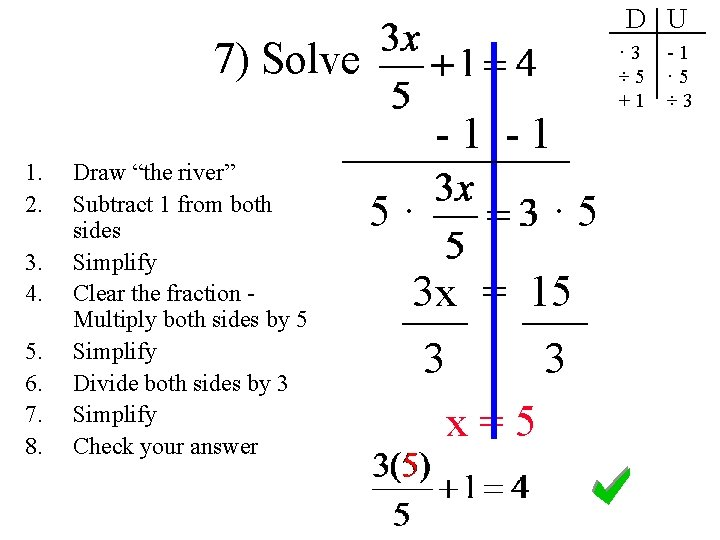D U 7) Solve -1 -1 1. 2. 3. 4. 5. 6. 7. 8. Draw “the river” Subtract 1 from both sides Simplify Clear the fraction Multiply both sides by 5 Simplify Divide both sides by 3 Simplify Check your answer 5· · 5 3 x = 15 3 3 x=5 · 3 ÷ 5 +1 -1 · 5 ÷ 3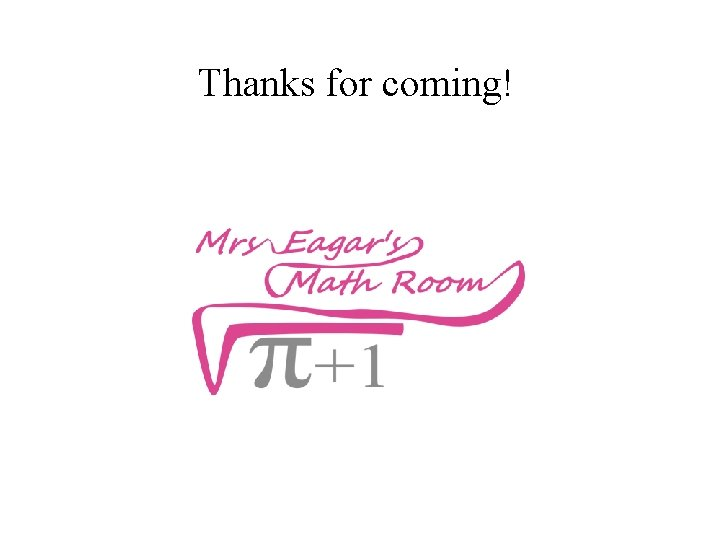Thanks for coming!